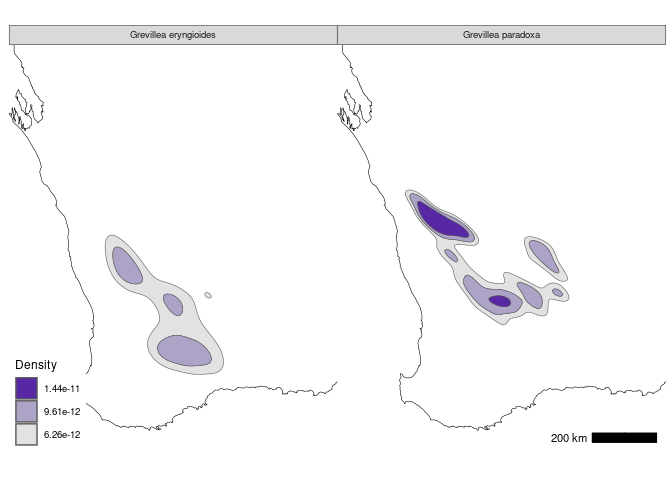# Kernel density estimates for tidy and geospatial data in the eks package

## Introduction

Kernel smoothers are essential tools for data analysis due to their ability to convey complex statistical information with concise graphical visualisations. The most widely used kernel smoother is the kernel density estimator (KDE), though there remain some important gaps in the implementation in R for specialised data types, most notably for tibbles (tidy data) within the tidyverse, and for simple features (geospatial data) within Geographical Information Systems (GIS) analysis. The tidy_kde and st_kde functions in the eks package fills in these gaps.

## Tidy data example

The data set we focus on is the crabs data set from the MASS package, with the variables FL frontal lobe size (mm), CW carapace width (mm) and sp species (B for blue, O for orange).

library(eks)
library(colorspace)
library(ggplot2)
library(dplyr)

## crabs data
data(crabs, package="MASS")
crabs2 <- select(crabs, FL, CW)
xlab <- "Frontal lobe size (mm)"
ylab <- "Carapace width (mm)"

The KDE for tidy data is computed by tidy_kde. From the output, the scatter plot of the data is generated by geom_point_ks and the contour plot of the KDE by geom_contour_ks. The bimodal structure of the data distribution, corresponding to the two species, is clearly visible from the KDE plot from tidy_kde. This is due to the optimal choice of the matrix of smoothing parameters. This optimal smoothing matrix is the plug-in bandwidth computed by ks::Hpi, and it is suitable for a wide range of data sets. For further details of the computation of the kernel density estimate and the bandwidth, see ?ks::kde and ?ks::Hpi.

## KDE contour plot + scatter plot
tkde2 <- tidy_kde(crabs2)
gkde2 <- ggplot(tkde2, aes(x=FL, y=CW)) + labs(x=xlab, y=ylab)
gkde2 + geom_point_ks(colour=8) + geom_contour_ks(colour=1)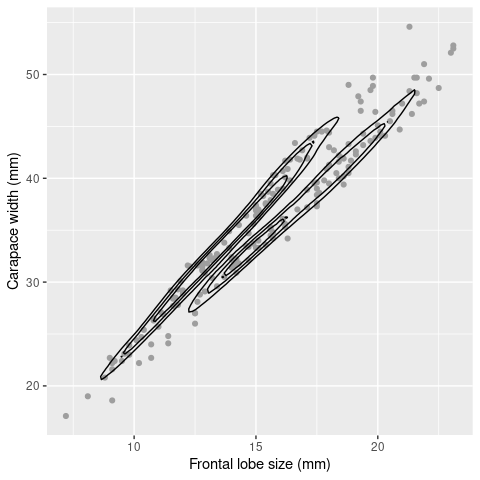On the other hand, the default bandwidth and the resulting KDE computed by ggplot2::geom_density_2d leads to an oversmoothed KDE which does not reveal the data bimodality.

## geom_density_2d KDE contour plot + scatter plot
mkde2 <- ggplot(crabs2, aes(x=FL, y=CW))
mkde2 + geom_point(colour=8) + geom_density_2d(colour=1, bins=4)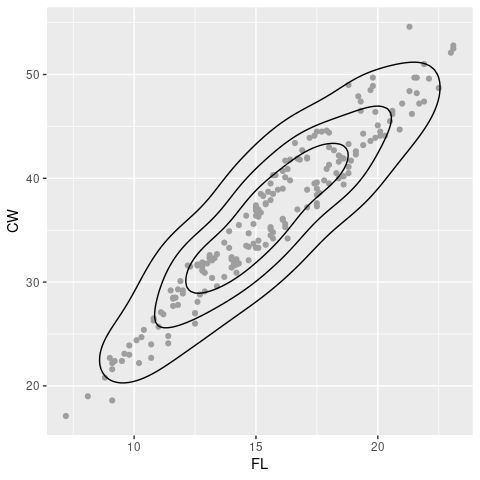The default choice of the contour levels in the eks package is based on probability contours. Probability contours offer an intuitive approach to selecting the contour levels that reveal the pertinent characteristics of the data distribution. See Chacon & Duong (2018, Chapter 2.2). Filled contour plots, generated by geom_contour_filled_ks, can be coloured with an appropriate sequential colour scale. For example, a 25% contour region (dark purple region) is the smallest region that contains 25% of the probability mass defined by the KDE. The 50% contour region consists of the union of the light purple region and the dark purple region, and it contains 50% of the data points etc. Note that the 25% and 50% contour regions of the crabs KDE are composed of separate, unconnected contour sub-regions.

## KDE filled contour plot
gkde2 + geom_contour_filled_ks(colour=1) + scale_fill_discrete_sequential(h1=275)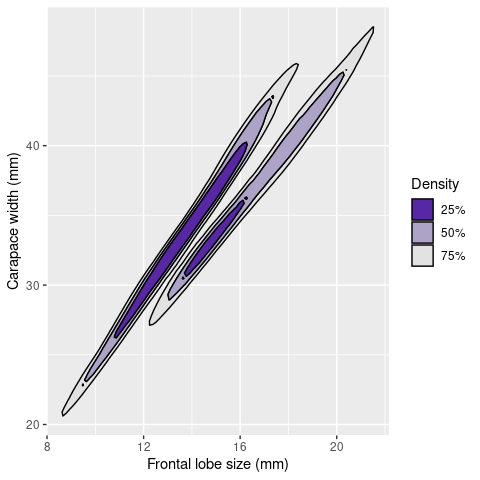As an alternative to these discretised contours, the usual ggplot2::geom_raster generates a plot with a continuous colour scale.

## KDE continuous colour plot
ggplot(tkde2, aes(x=FL,y=CW)) + geom_raster(aes(fill=estimate), interpolate=TRUE) +
labs(x=xlab, y=ylab) + scale_fill_continuous_sequential(h1=275)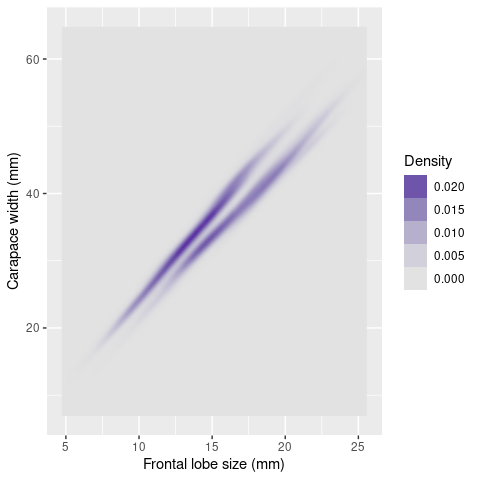One of the main advantages of ggplot2 is its ability to handle multiple related plots, in this case, KDE plots for each species. The KDE with blue contours is for the B species, and orange contours for the O species.

crabs2g <- select(crabs, FL, CW, sp)
crabs2g <- group_by(crabs2g, sp)
tkde2g <- tidy_kde(crabs2g)
gkde2g <- ggplot(tkde2g, aes(x=FL, y=CW, group=sp)) + labs(x=xlab, y=ylab,
colour="Species") + scale_colour_manual(values=c(4, 7))

## superposed KDE contour plots + scatter plots
gkde2g + geom_point_ks(colour=8) +
geom_contour_ks(aes(colour=sp)) + guides(colour=guide_legend(title="Species"))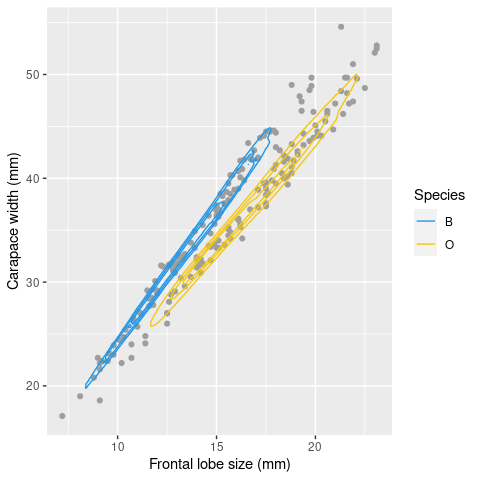We can also display each species KDE on its own set of axes.

## facetted KDE contour plots + scatter plots
gkde2g + geom_point_ks(colour=8) +
guides(colour=guide_legend(reverse=FALSE)) +
geom_contour_ks(aes(colour=sp)) + facet_wrap(~sp)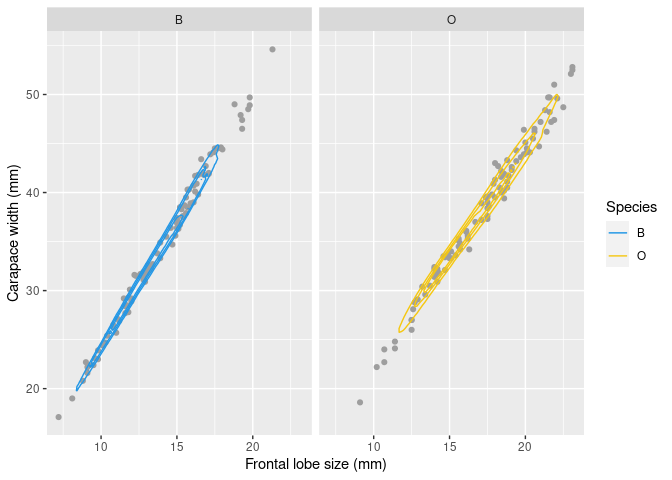The probability contour levels computed in geom_contour_ks and geom_contour_filled_ks are relative to the grouping variable. So whilst the same probability 25% level is applied to both groups KDE, the height of 25% contour region for the blue species is 0.0414, and for the orange species it is 0.0318. For a direct comparison, it is useful to have a set of fixed contour heights for all KDEs . A heuristic solution is implemented in contour_breaks. For the crabs data, this gives 0.0142, 0.0264, 0.0414. Since the KDE for the B species exceeds the highest level 0.0414, whereas the O KDE doesn’t reach this is level, the former KDE is more peaked.

## facetted KDE filled contour plots with fixed contour levels for all facets
bkde2g <- contour_breaks(tkde2g)
gkde2g + geom_contour_filled_ks(breaks=bkde2g, colour=1) +
scale_fill_discrete_sequential(h1=275) + facet_wrap(~sp)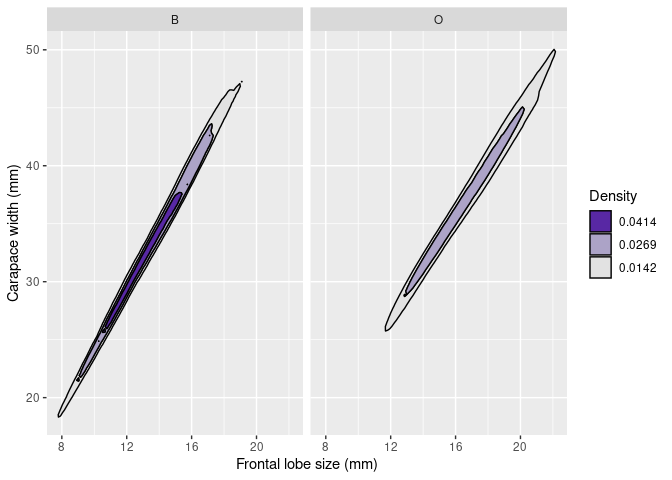## Geospatial data example

GIS for geospatial data analysis in R is implemented in the sf package, and the eks package builds on this. To illustrate geospatial KDE, we focus on the grevilleasf data set in the eks package. It has 22303 rows, where each row corresponds to an observed grevillea plant in Western Australia. In addition, we utilise wa, the geospatial polygon for Western Australia. Both of these geospatial data sets are in the EPSG:7850 (GDA2020/MGA zone 50) projection.

library(sf)

## Grevillea data
data(grevilleasf, package="eks")
grevilleasf <- mutate(grevilleasf, species=factor(species))
eryngioides <- filter(grevilleasf, name %in% "Grevillea eryngioides")
grevillea_ep <- filter(grevilleasf, name %in% c("Grevillea eryngioides",
grevillea_ep <- group_by(grevillea_ep, name)
xlim <- c(165479.3, 1096516.3); ylim <- c(6101931, 7255991)

## WA polygon
data(wa, package="eks")
gwa <- geom_sf(data=wa, fill=NA, colour=1)

Since geospatial data can be visualised with both base R and ggplot2 graphics engines, we provide code for both: their outputs are similar due to the standardisation of geospatial maps within GIS. Though these plots can’t be mixed due to fundamental differences between the graphical rendering in base R and ggplot2. This is the reason that, for example, adding a scale bar requires functions from different packages.

## base R scatter plot
plot(st_geometry(wa), xlim=xlim, ylim=ylim)
pal=c(3,6), pt_pch=16:17, pt_cex=c(1,1), title="Species",
pos="topright")
mapsf::mf_scale(size=200, lwd=4)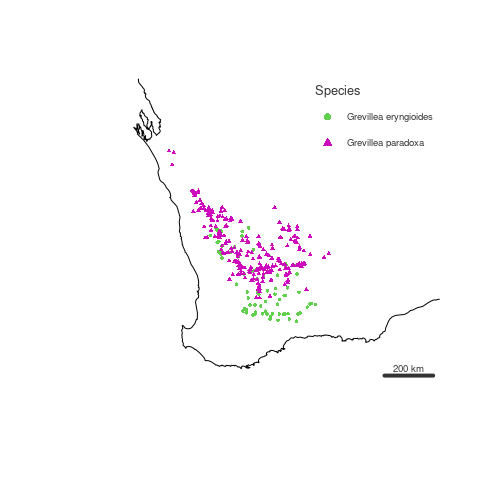## geom_sf scatter plot
location="br", width_hint=0.2, bar_cols=1)
theme_set(ggthemes::theme_map())
theme_update(legend.position=c(0.99,0.99), legend.justification=c(1,1))
ggplot() + gwa + gsc +
geom_sf(data=grevillea_ep, aes(colour=name, shape=name), size=0.5) +
coord_sf(xlim=xlim, ylim=ylim) + scale_colour_manual(values=c(3, 6)) +
guides(colour=guide_legend(title="Species"), shape=guide_legend(title="Species"))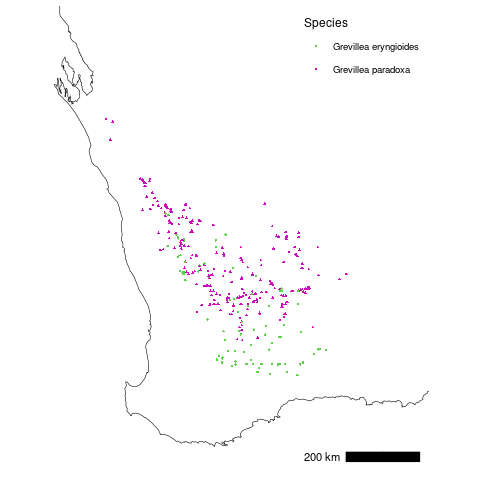The KDE for geospatial data is computed by st_kde. The calculations of the KDE, including the bandwidth matrix fo smoothing parameters, are the same as in tidy_kde. Though, unlike for tidy_kde where the probability contour regions are computed dynamically in geom_contour_filled_ks, the 1% to 99% regions are explicitly computed as multipolygons in st_kde since this conversion can be computationally heavy to execute for each plot. For display, it is a matter of selecting the appropriate contour regions. The quartile contours 25%, 50%, 75% are selected by default in geom_contour_filled_ks for tidy data. This is also the case for the base R plot.

skde1 <- st_kde(paradoxa)

## base R contour plot
plot(st_geometry(wa), xlim=xlim, ylim=ylim)
mapsf::mf_scale(size=200, lwd=4)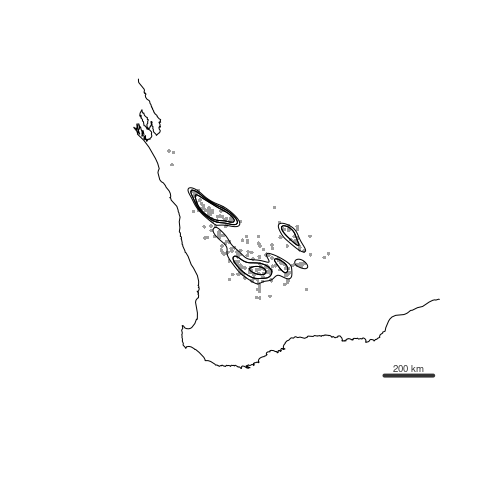On the other hand, we can’t replicate exactly the default contour selection in geom_sf, so we first apply the auxiliary function st_get_contour to the input of geom_sf.

## geom_sf contour plot
gs <- ggplot(skde1) + gwa + gsc
gs + geom_sf(data=paradoxa, col=8, size=0.5) +
geom_sf(data=st_get_contour(skde1), colour=1, fill=NA, show.legend=FALSE) +
coord_sf(xlim=xlim, ylim=ylim) + ggthemes::theme_map()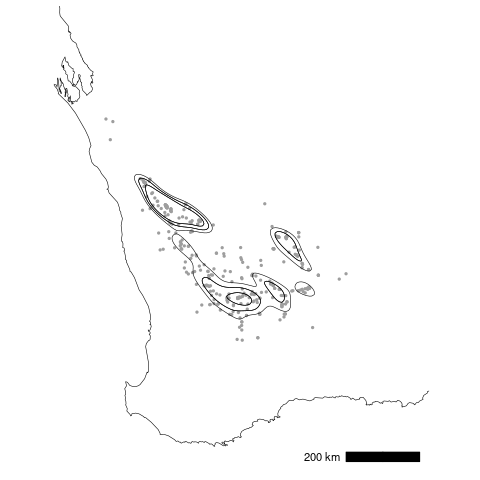To generate a filled contour plot, the only required changes are to input an appropriate colour scale function, and for a base R plot, to set legend=TRUE since its default value is FALSE.

## R base filled contour plot
plot(st_geometry(wa), xlim=xlim, ylim=ylim)
plot(skde1, add=TRUE, pal=function(.) { sequential_hcl(n=., h1=275, rev=TRUE) })
mapsf::mf_scale(size=200, lwd=4)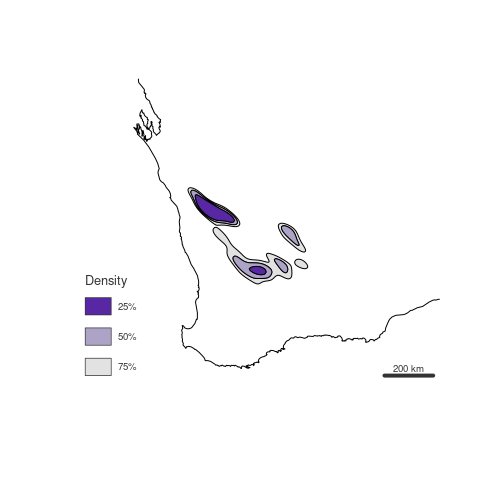## geom_sf filled contour
gs + geom_sf(data=st_get_contour(skde1), aes(fill=label_percent(contlabel))) +
scale_fill_discrete_sequential(h1=275)  +
coord_sf(xlim=xlim, ylim=ylim) + ggthemes::theme_map()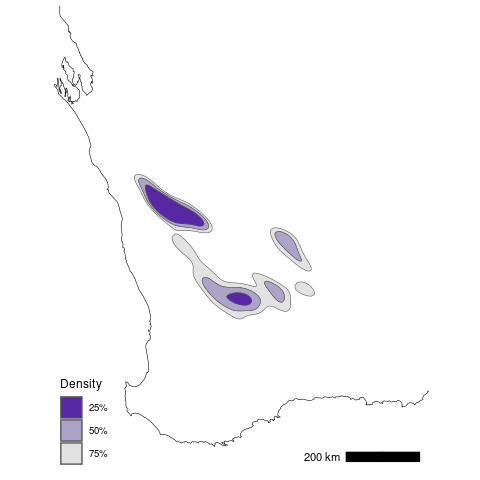Since the output from st_kde is compatible with geom_sf, then it is easy to generate multiple maps of related geospatial KDEs. For example, KDEs for each Grevillea species, with probability contour levels or with fixed contour levels:

skde1g <- st_kde(grevillea_ep)

## facetted geom_sf filled contour
gsg <- ggplot(skde1g) + gwa + gsc
gsg + geom_sf(data=st_get_contour(skde1g), aes(fill=label_percent(contlabel))) +
scale_fill_discrete_sequential(h1=275) +
facet_wrap(~name) + coord_sf(xlim=xlim, ylim=ylim) + ggthemes::theme_map()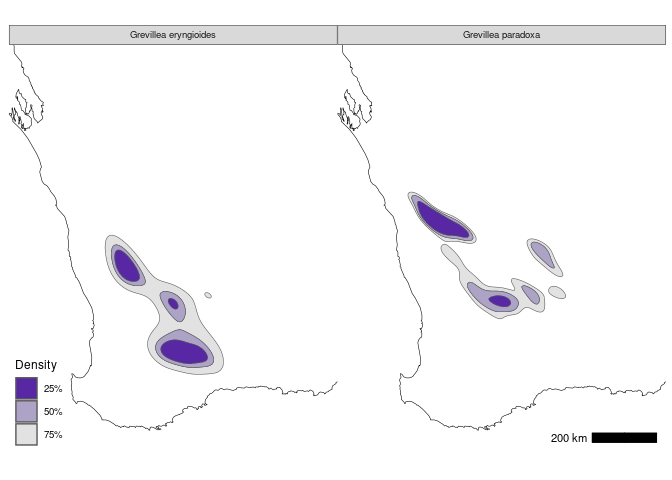## facetted geom_sf filled contour with fixed contour levels for all facets
bkde1g <- contour_breaks(skde1g)
gsg + geom_sf(data=st_get_contour(skde1g, breaks=bkde1g), aes(fill=contlabel)) +
scale_fill_discrete_sequential(h1=275) + facet_wrap(~name) +
coord_sf(xlim=xlim, ylim=ylim) + ggthemes::theme_map()